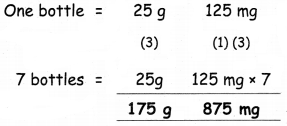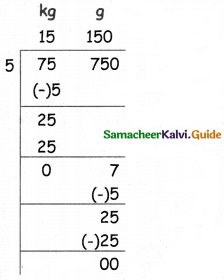Students can download 5th Maths Term 2 Chapter 4 Measurements Ex 4.1 Questions and Answers, Notes, Samacheer Kalvi 5th Maths Guide Pdf helps you to revise the complete Tamilnadu State Board New Syllabus, helps students complete homework assignments and to score high marks in board exams.

## Tamilnadu Samacheer Kalvi 5th Maths Solutions Term 2 Chapter 4 Measurements Ex 4.1

Question 1.
Fill in the blanks.

(i) 7 kg 400 g = ______ g
7400

(ii) 5 g 50 mg = ______ mg
5050(iii) 9500 mg = ______ g ______ mg
9,500

(iv) 15 kg 350 g = ______ g
15350

(v) 6250 g = ______ kg ______ g
6,250Question 2.

(i) 4 kg 250 g + 3 kg 450 g4 kg 250 g + 3 kg 450 g = 7 kg 700 g

(ii) 75g 430 mg + 750 g75g 430 mg + 750 g = 825 g 430 mg(iii) 97 kg 45 g + 77 kg 450g + 33 kg 250 g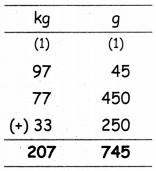97 kg 45 g + 77 kg 450g + 33 kg 250 g = 207 kg 745 g

(iv) 75 kg 400 g + 30 kg 250 g75 kg 400 g + 30 kg 250 g = 105 kg 650 gQuestion 3.
Subtract the following:

(i) 40 kg 350 g – 25 kg 200 g40 kg 350 g – 25 kg 200 g = 15 kg 150 g

(ii) 35 kg 850 g – 18 kg 500 g35 kg 850 g – 18 kg 500 g = 17 kg 350 g(iii) 985 kg 475 g – 275 kg 325 g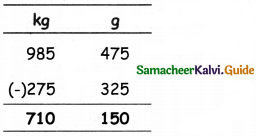985 kg 475 g – 275 kg 325 g = 710 kg 150 g

(iv) 700 kg – 300 kg 500 g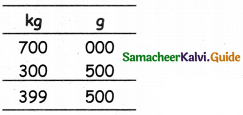700 kg – 300 kg 500 g = 399 kg 500 gQuestion 4.
Multiply the following:

(i) 4 kg 300 g × 7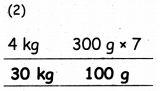4 kg 300 g × 7 = 30 kg 100 g

(ii) 17kg 750 g × 8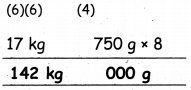17kg 750 g × 8 = 142 kg(iii) 25 kg 550 g × 4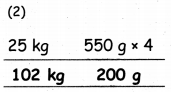25 kg 550 g × 4 = 102 kg 200 g

(iv) 72g 350 mg × 5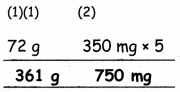72g 350 mg × 5 = 361 g 750 mgQuestion 5.
Divide the following:

(i) 99 kg 99 0g ÷ 3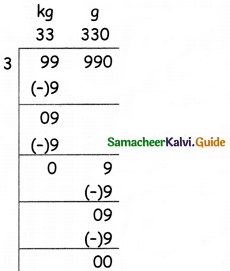99 kg 99 0g ÷ 3 = 33 kg 330 g

(ii) 147 g 630 mg ÷ 7147 g 630 mg ÷ 7 = 21 g 90 mg(iii) 550 kg 220 g ÷ 11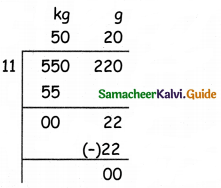550 kg 220 g ÷ 11 = 50 kg 20 g

(iv) 484 g 384 mg ÷ 4484 g 384 mg ÷ 4 = 121 g 96 gQuestion 6.
What is the total weight of 7 kg 500 g of cashew nut and 3 kg 350 g of pista?
cashew nut = 7 kg 500 g
pista = 3 kg 350 g
Total weight = 7 kg 500 g + 3 kg 350 g
= 10 kg 850 gQuestion 7.
Vimal had a sack of cotton seeds weighing 50 kg 350 g. He used 7 kg 300 g cotton seeds to feed his cow. How much cotton seed will be remaining after feeding his cow?Remaining cotton seeds = 43 kg 50 gQuestion 8.
A glass bottle can contain 25 g 125 mg of medicine, how much medicine can 7 such bottles contain?